TitleCollege Algebra
Tutorial 15: Equations with Rational ExpressionsLearning Objectives

 After completing this tutorial, you should be able to: Solve equations with rational expressions. Know if a solution is an extraneous solution or not.Introduction

 The equations that we will be working with in this section all have rational expressions (fractions - yuck!).  After a few magical steps, we can transform these equations with rational expressions into linear equations.  From there, you solve the linear equation like you normally do.  If you need a review on how to solve a linear equation, feel free to go to Tutorial 14: Linear Equations in One Variable. No matter what type of equation you are working with in this section, the ultimate goal is to get your variable on one side and everything else on the other side using inverse operations.Tutorial

 Solving Rational Equations

Step 1: Simplify by removing the fractions.

 We do this by multiplying both sides by the LCD.  If you need a review on finding the LCD of a rational expression, go to Tutorial 10: Adding and Subtracting Rational Expressions. Note that even though this is not the same as adding and subtracting rational expressions, you still find the LCD in the same manner. So if you go to this link, just look at finding the LCD, NOT adding and subtracting rational expressions.

Step 2: Solve the remaining equation.

 In this tutorial the remaining equations will all be linear.  If you need a review on solving linear equations go back to Tutorial 14: Linear Equations.

Step 3: Check for extraneous solutions.

 For rational equations, extraneous solutions are values that cause any denominator in the original problem to be 0.  Of course, when we have 0 in the denominator we have an expression that is undefined.  So, we would have to throw out any values that would cause the denominator to be 0.

In Tutorial 14: Linear Equations, I told you that when you multiply both sides by the same constant that the two sides would remain equal to each other. But we can not guarantee that if you are multiplying by an expression that has the variable you are solving for - which is the situation we will be running into in this section.  Sometimes this will cause extraneous solutions.Example 1:  Solve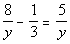for y.

 Step 1: Simplify by removing the fractions.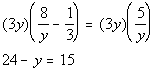Step 2: Solve the remaining equation.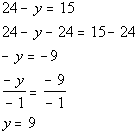*Inverse of add. 24 is sub. 24   *Inverse of mult. by -1 is div. by -1

 Step 3: Check for extraneous solutions.

 Note that 9 does not cause any denominators to be zero.  So it is not an extraneous solution. 9 is the solution to our equation.Example 2:  Solve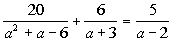for a.

 Step 1: Simplify by removing the fractions.*Factor 1st den.     *Mult. both sides by LCDof (a + 3)(a - 2)

 Step 2: Solve the remaining equation.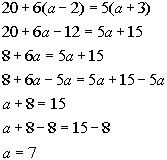*Remove ( ) by using dist. prop.   *Get all a terms on one side   *Inverse of add. 8 is sub. 8

 Step 3: Check for extraneous solutions.

 Note that 7 does not cause any denominators to be zero.  So it is not an extraneous solution. 7 is the solution to our equation.Example 3:  Solve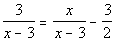for x.

 Step 1: Simplify by removing the fractions.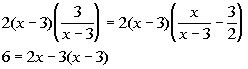*Mult. both sides by LCD of 2(x - 3)

 Step 2: Solve the remaining equation.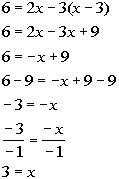*Remove ( ) by using dist. prop.   *Inverse of add. 9 is sub. 9   *Inverse of mult. by -1 is div. by -1

 Step 3: Check for extraneous solutions.

 Note that 3 does cause two of the denominators to be zero.  So 3 is an extraneous solution.  That means there is no solution. The answer is NO solution.Practice Problems

 These are practice problems to help bring you to the next level.  It will allow you to check and see if you have an understanding of these types of problems. Math works just like anything else, if you want to get good at it, then you need to practice it.  Even the best athletes and musicians had help along the way and lots of practice, practice, practice, to get good at their sport or instrument.  In fact there is no such thing as too much practice. To get the most out of these, you should work the problem out on your own and then check your answer by clicking on the link for the answer/discussion for that  problem.  At the link you will find the answer as well as any steps that went into finding that answer.Practice Problems 1a - 1b: Solve the given equation.

 1a.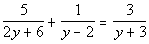(answer/discussion to 1a) 1b.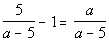(answer/discussion to 1b)Need Extra Help on these Topics?

The following is a webpage that can assist you in the topics that were covered on this page:

 http://www.sosmath.com/algebra/solve/solve0/solve0.html#fraction Problems 1 and 2 of this part of the webpage helps with solving fractional equations that lead to linear equations.  ONLY do problems 1 and 2.

Go to Get Help Outside the Classroom found in Tutorial 1: How to Succeed in a Math Class for some more suggestions.

WTAMU > Virtual Math Lab > College Algebra

Videos at this site were created and produced by Kim Seward and Virginia Williams Trice.
Last revised on Dec 16, 2009 by Kim Seward.